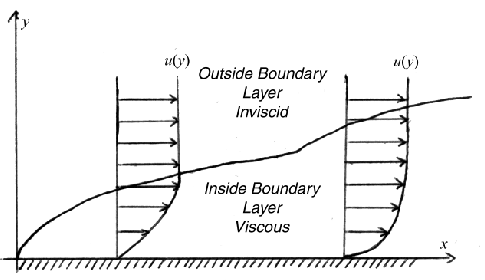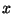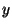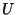Next: Boundary Layer Parameters Up: Boundary Layers: An Aerospace Previous: Boundary Layers: An Aerospace

Subsections

# Introduction

Before this century, there were two schools of thought in fluid dynamics. One school focused on ideal flows. The ideal flows were governed by simple, linear equations that mathematicians already knew a great deal about. The solutions obtained with ideal flows were somewhat accurate, but failed to predict phenomena such as drag.

The other school of thought was concerned with establishing the nature of the interactions among fluid particles. Those of this school noted that when nearby fluid particles had different velocities, the particles tended to exchange momentum so that their velocities became more similar. This property, called viscosity, was completely ignored in ideal flow solutions. Unfortunately, the equations describing viscous interactions were very complex and analytically intractable.

The question people asked was whether to focus on ideal flows, which ignore viscosity, and thus yield less than accurate solutions, or to focus on viscous flows, which had equations so complex no one could solve them. Fluid dynamicists had a dilemma.In 1904, Ludwig Prandtl (Figure 1) changed that. He noted that, far away from a solid wall, viscous interactions were not that significant in determining the flow field. However, in a thin region near a solid boundary, the viscous interactions had a significant effect on fluid motion. Prandtl's idea of a boundary layer, a thin region near a surface where all of the viscous effects reside, made tractable the flow calculations that take viscosity into account.

## Definition of a Boundary LayerA boundary layer is a thin region of fluid near a wall where viscous effects are important in determining the flow field. The boundary layer is a buffer region between the wall below and the inviscid free-stream above. Mathematically, its main purpose is to allow an inviscid flow solution to satisfy the no-slip condition at the wall.

Figure 2 shows a boundary layer. Conventionally, the-axis is parallel to the wall, while the-axis is perpendicular to the wall and cuts right through the boundary layer. At any given-coordinate, we can draw the velocity distribution as a function of. This is the most common way to illustrate a boundary layer.

Figure 2 shows velocity cross sections of two points in the boundary layer. The first is cross section for a laminar boundary layer; the second is after transition and represents a turbulent boundary layer. Note that, in a boundary layer, the velocity is always zero at the wall, and asymptotically approaches the free-steam velocity (denoted by) at infinity.

The boundary layer typically grows in thickness in the streamwise direction. Turbulent boundary layers grow faster than laminar ones.

## Importance to Engineering Applications

Solutions of the full Navier-Stokes equations are expensive. The equations are highly nonlinear, of second order, and, for subsonic flows, elliptic in space.

Inviscid solutions are very cheap compared to Navier-Stokes solutions. By assuming that all of the viscosity in the flowfield resides in a thin, viscous boundary layer, we are free to solve the rest of the flowfield using an inviscid solution.

The solution of the flow inside the boundary layer is cheaper as well. By assuming a thin boundary layer, several terms become negligible (see Section 3), and the elliptic equations become parabolic. This allows solution techniques that march in one direction from a starting point, as opposed to considering the whole flow field at every point.

Even as full Navier-Stokes solutions become less and less expensive, the boundary layer concept retains importance for several reasons.

The boundary layer solutions are less expensive still. In some cases, accurate flowfield solutions can be obtained in seconds. These solutions are accurate enough for many purposes; full Navier-Stokes solutions are unnecessary in these situations.

Even when more accurate solutions are needed, a good boundary layer solution can serve as an initial flowfield for an iterative Navier-Stokes code. Iterative codes converge much faster when they have good initial guesses.

Turbulence is the great pest of computational fluid dynamics today. Boundary layer equations, however, handle turbulence much more easily. This stems from the fact that general turbulence models, as are used in many Navier-Stokes codes, are not yet very successful. However, turbulence models used in boundary layers are successful, because the nature of wall turbulence is relatively well understood.

Finally, Navier-Stokes codes often solve flows in a black-box manner, i.e., outputting massive tables of numbers for whatever problem one gives it. However, boundary layer solutions are specialized to a particular sort of flow, which can help a human to understand the nature of the flow better. For example, if an airfoil experiences a separation problem, a boundary layer solution may be more useful to an engineer trying to diagnose the problem, because he or she is more intimate with the data and the equations used to calculate them. Extracting this sort of information from a table may be difficult or impossible.Next: Boundary Layer Parameters Up: Boundary Layers: An Aerospace Previous: Boundary Layers: An Aerospace
Carl Banks
1999-12-23